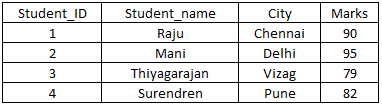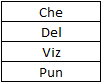# SQL – MID()

#### Prev     Next

SQL MID() function extracts the characters from a text field. Syntax for SQL MID() function is given below.

# SQL Syntax for MID() function:

 Syntax for MID() function in SQL SELECT MID(column_name, start, length) FROM table_name;

Where,

• Column_name – It is the column name from which we need to extract the characters. It’s a mandatory field.
• Start – This is the starting position from where the characters need to be extracted. It’s a mandatory field and 1 is the starting character
• Length – Number of characters to be extracted. If it is left empty, it will return all characters till the end of the text. It’s an optional field.

Please consider the following table with few records as given below.

Table name (for example): student
Column names in this table: Student_ID, Student_name, City and Marks
Available records: 4 rows# Example: how to use SQL MID() function:

To extract only first 3 characters from the column ‘City’, please execute below query.

# SQL query:

SELECT MID(City, 1, 3) from student;

# SQL query output:# List of other inbuilt functions in SQL:

Please click on each SQL functions below to know more about them and sample SQL queries with output.

 1. Aggregate functions SUM() SQL SUM() function returns the sum of a column. The column should be numeric. COUNT() SQL COUNT() function returns/counts the number of rows in a query. But, it will not count any null values/column. AVG() SQL AVG() function returns the average value of a column. The column should be numeric. MIN() SQL MIN() function returns the minimum or smallest value of a column. The column should be numeric. MAX() SQL MAX() function returns the maximum or biggest value of a column. The column should be numeric. FIRST() SQL FIRST() function returns the first value of the given column. LAST() SQL LAST() function returns the last value of the given column. 2. Scalar functions LEN() SQL LEN() function returns the total length of the given column. MID() SQL MID() function extracts the characters from a text field. ROUND() SQL ROUND() function is used to round a numeric value to the nearest integer or to the number of decimals specified by the user. LCASE() SQL LCASE() function converts the given column to lowercase. UCASE() SQL UCASE() function converts the given column to uppercase. NOW() SQL NOW() function is used to return system’s current date & time. FORMAT() SQL FORMAT() function is used to format a field/column value how it should be displayed in the output. SQRT() SQL SQRT() function is used to find square root value of the given number. RAND() SQL RAND() function is used to generate some random numbers. CONCAT() SQL CONCAT() function is used to concatenate 2 or more strings and forms a single string. 3. Group by function GROUP BY() GROUP BY() function is used in conjunction with a SELECT statement and aggregate functions (such as SUM(), AVG(), MAX() etc.), to group similar data in result-set by one or more columns. 4. Having function Having() HAVING() function in SQL acts as a filter which is used to specify conditions on aggregate functions in WHERE clause. The main purpose of HAVING() function is, aggregate functions such as SUM(), COUNT(), AVG(), etc. can’t be used in where clause. So, they are used in where clause with the help of HAVING() function.